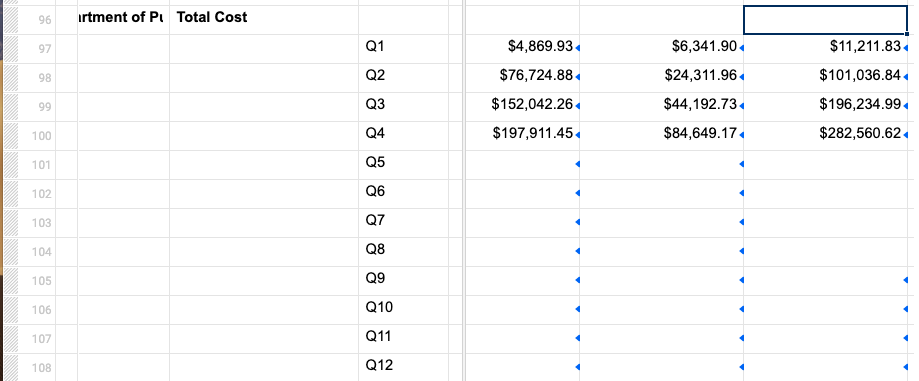# Comparing the most recent two quarters

Hi,

I have sheet collecting total cost dollar figures as they are entered each quarter (over a three year period, so 12 quarters total) into another sheet.

The accounting system is accrual based, so each quarter the dollar amount should/must increase. I'm trying to figure out a formula to automatically compare the most recent quarter entered and compare it with the previous quarter to indicate if the amount in the most recent quarter decreased. My plan was to indicate this in the parent row (total cost), but I can't figure out the best way to create a formula that will recognize the most recent quarter and compare it with the previous. I attached a screenshot so you can see how the sheet is setup.

Thanks for the help,

BrettTags:

• This can actually be done with a pretty straightforward solution. How were you intending to display the results? An actual dollar amount? An up arrow vs a down arrow? Something else?

• Initially I thought it would get trigger a flag on and off, but the column is set as text/numbers because the dollar figures so I'm not sure that would work. if it can't work, I was just going to have it say "Decreased."

However, right now I haven't thought of a way to automatically compare the two most recent without the formula becoming long and cumbersome, or me having to go in and switch the formula each quarter.

Thanks for the help.

• Ok. Give this a shot...

=IF(INDEX([Column Name]97:[Column Name]108, COUNTIFS([Column Name]97:[Column Name]108, ISNUMBER(@cell))) < INDEX([Column Name]97:[Column Name]108, COUNTIFS([Column Name]97:[Column Name]108, ISNUMBER(@cell)) - 1), "Decreased")

.

Basically we use an INDEX function to pull the data from a set range ([Column Name]97:[Column Name]108). To determine the row number for the INDEX function, we simply use a COUNTIFS function to count how many rows have numbers in them (within the same range). That will be your most recent quarter that has been filled out.

We then duplicate the INDEX formula and subtract 1 from the row number after getting the COUNTIFS number. This will pull the data from the cell ABOVE the most recent quarter (the quarter before). If the first number is less than the second number, then it will display "Decreased" (or whatever text you want it to).

## Help Article Resources

Want to practice working with formulas directly in Smartsheet?

Check out the Formula Handbook template!